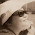## Thursday, 10 January 2013

### Primes (Notes, p2) Q1, Q2, Q3, Q7

The following statements are false.
Explain or illustrate with examples (if applicable) to show why they are false

Q1 All odd numbers are prime.

Q2 All prime numbers are odd.

Q3. All even numbers are composite.

Q7. A prime number is always smaller than a composite number.

Enter your response, label them neatly.
Remember to sign off with your group number.

1.Group 3
I) no, 1 is an odd number but it is not a prime number but is a unit.
II) no, 2 is a prime number but it is an even number
III) no, 2 is a prime number but it is a even number
VII) no, 7, which is a prime number is larger than 4 which is a composite number

1.Q1: Correct
Q2: Correct
Q4: Correct
Q7: Correct

Score: 4 points

2.Q1. Because number 9 is not a prime number as it has more than 2 factors

Q2. Number 2 is even and is a prime number

Q3. No. Number 2 is a prime number and is even

Q7. You can compare the largest prime number with the smallest composite number.

1.Post by group 1:)

2.Q1: Correct. Good to enforce the fact that "9 is an odd number"
Q2: Correct
Q4: Correct
Q7: Elaborate or illustrate with an example.

Score: 3 points

3.This comment has been removed by the author.

4.This comment has been removed by the author.

5.Q1 All odd numbers are prime.
A1 False. 9 is a odd number but it is a composite

Q2 All prime numbers are odd.
A2. False. 2 is a prime number which is also a even number

Q3. All even numbers are composite.
A3. False. 2 which is a even number is a prime.

Q7. A prime number is always smaller than a composite number.
Q7. False. A prime number like 17 is greater than 4 which is a composite

1.Q1: Correct
Q2: Correct
Q4: Correct
Q7: Correct

Score: 4 points

2.What's the Group number?